# How to Calculate and Solve for the Total Surface Area of a Conical Frustum | The Calculator Encyclopedia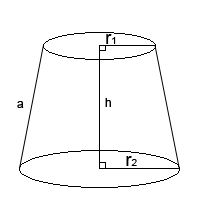The image above is a conical frustum.

To compute the total surface area of a conical frustum requires three essential parameters which are the radius of the lower base (R), radius of the upper base (r) and the height (h).

The formula for calculating the total surface area of a conical frustum;

A = π[R² + r² + (R + r)√((R – r)² + h²)]

Where;
A = Total surface area of the conical frustum
R = Radius of the lower base
r = Radius of the upper base
h = Height of the conical frustum

Let’s solve an example;
Find the total surface area of the conical frustum when the radius of the upper base is 11 cm, radius of the lower base is 17 cm and the height is 30 cm.

This implies that;
r = Radius of the upper base = 11 cm
R = Radius of the lower base = 17 cm
h = Height of the conical frustum = 30 cm

A = π[R² + r² + (R + r)√((R – r)² + h²)]
A = π[17² + 11² + (17 + 11)√((17 – 11)² + 30²)]
A = π[289 + 121 + (28)√((6)² + 900)]
A = π[289 + 121 + (28)√(36+ 900)]
A = π[289 + 121 + (28)√(936)]
A = π[289 + 121 + (28)(30.59)]
A = π[289 + 121 + 856.63]
A = π[1266.63]
A = 3979.25

Therefore, the total surface area of the conical frustum is 3979.25 cm².

Nickzom Calculator – The Calculator Encyclopedia is capable of calculating the total surface area of a conical frustum.

To get the answer and workings of the total surface area of a conical frustum using the Nickzom Calculator – The Calculator Encyclopedia. First, you need to obtain the app.

You can get this app via any of these means:

To get access to the professional version via web, you need to register and subscribe for NGN 1,500 per annum to have utter access to all functionalities.
You can also try the demo version via https://www.nickzom.org/calculator

Once, you have obtained the calculator encyclopedia app, proceed to the Calculator Map, then click on Mensuration under the Mathematics section.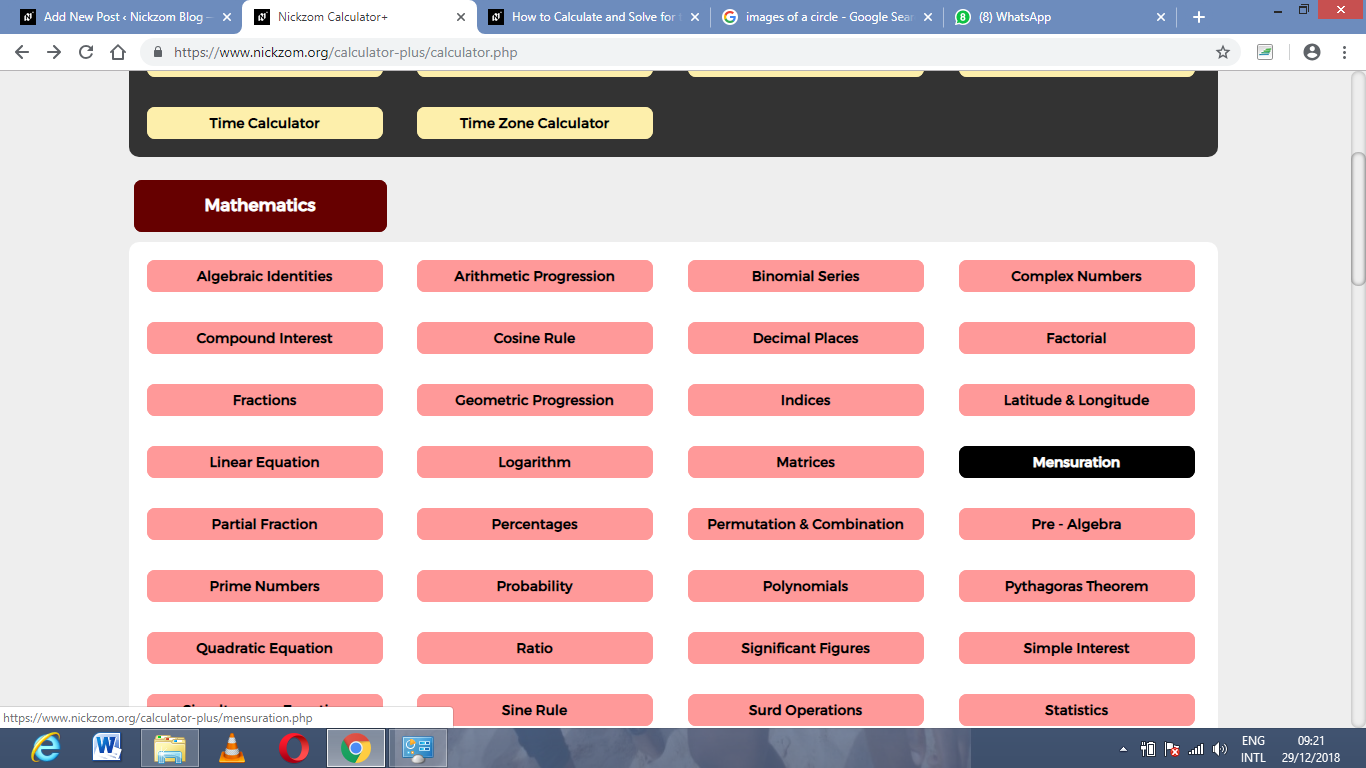Now, click on Total Surface area of a conical frustum under Mensuration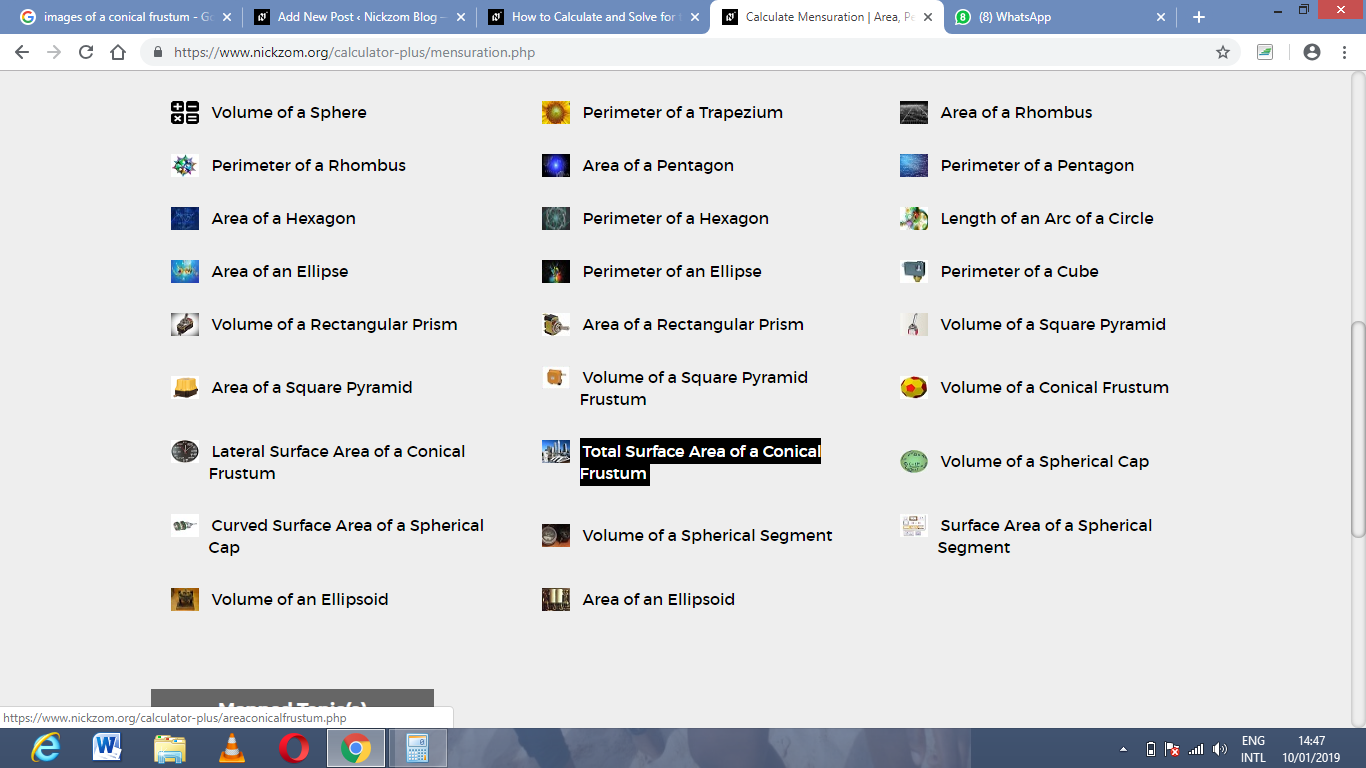The screenshot below displays the page or activity to enter your values, to get the answer for the total surface area of a conical frustum according to the respective parameters which are the radius of the lower base (R), radius of the lower base (r) and the height of the conical frustum.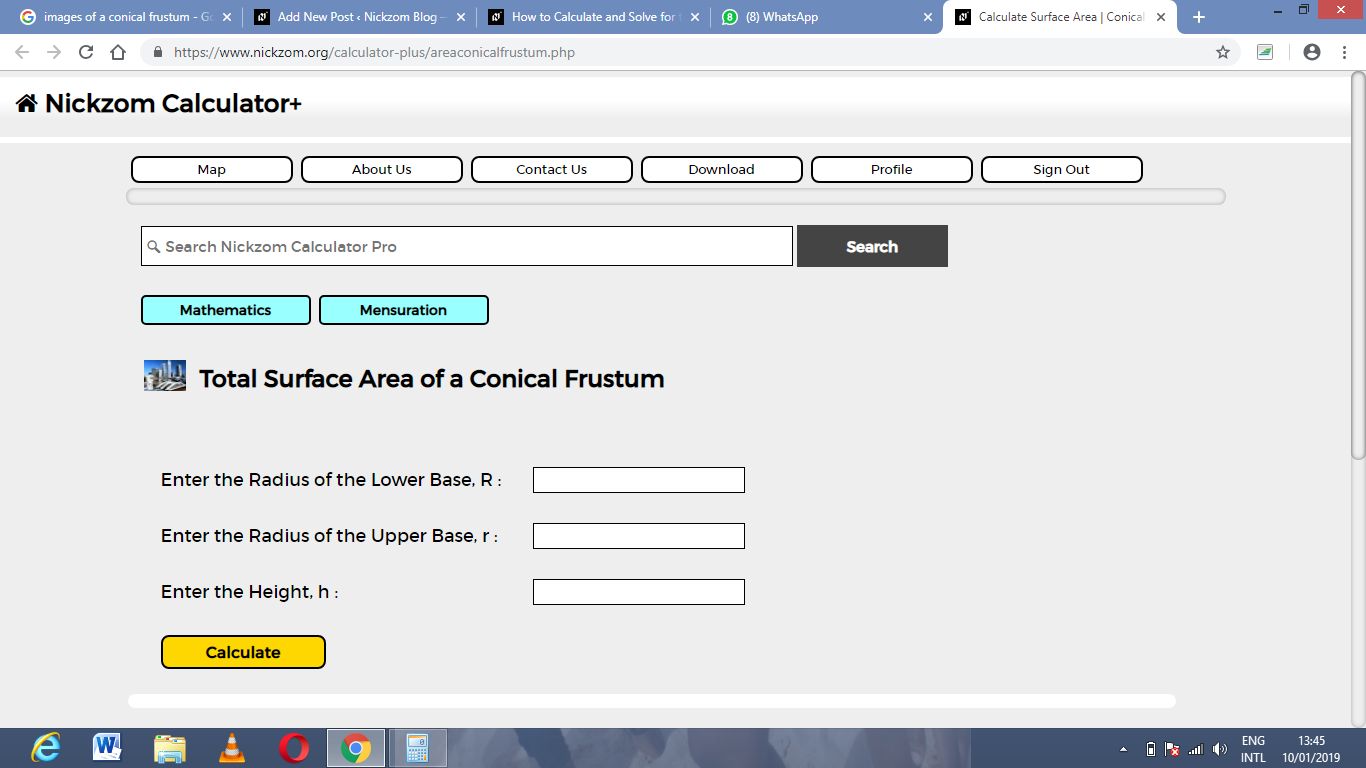Now, enter the values appropriately and accordingly for the parameters as required by the example above where the radius of the lower base is 17 cm, radius of the upper base is 11 cm and the height is 30 cm.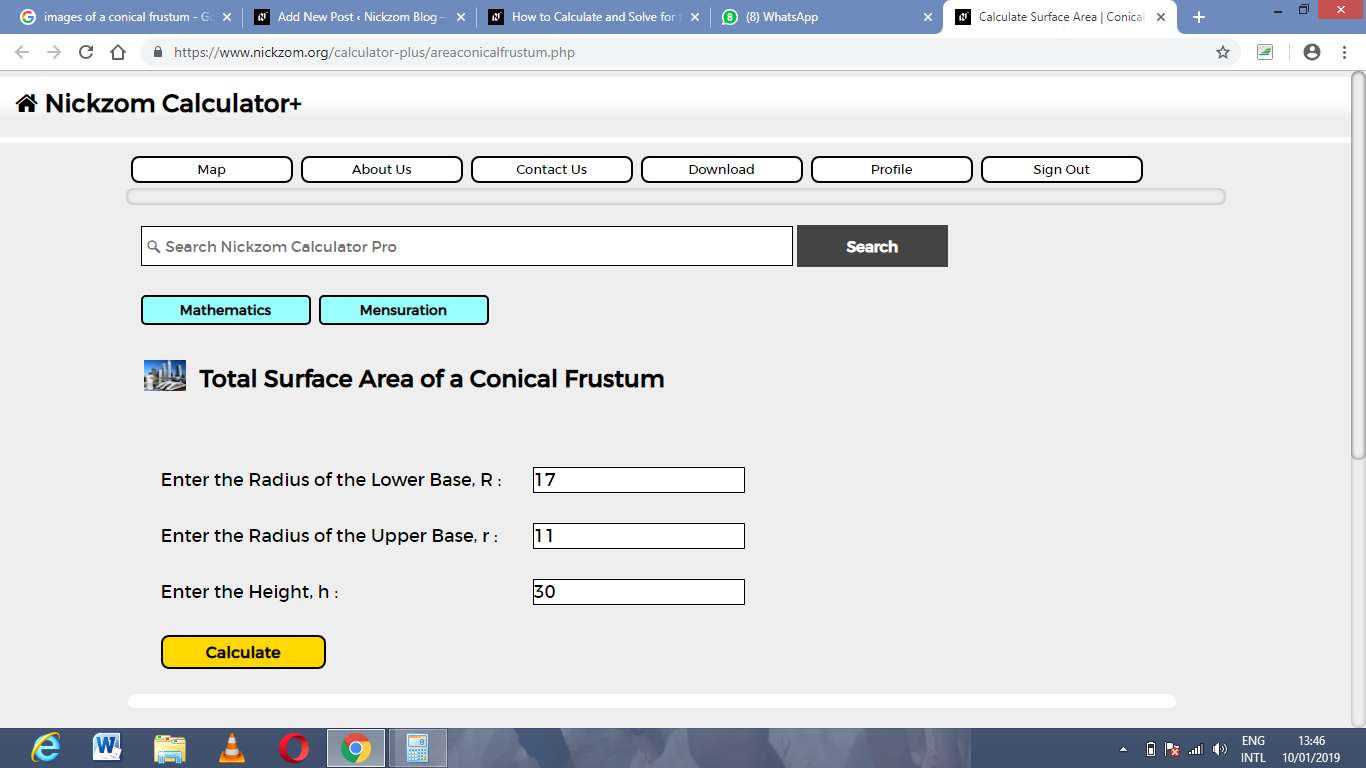Finally, Click on Calculate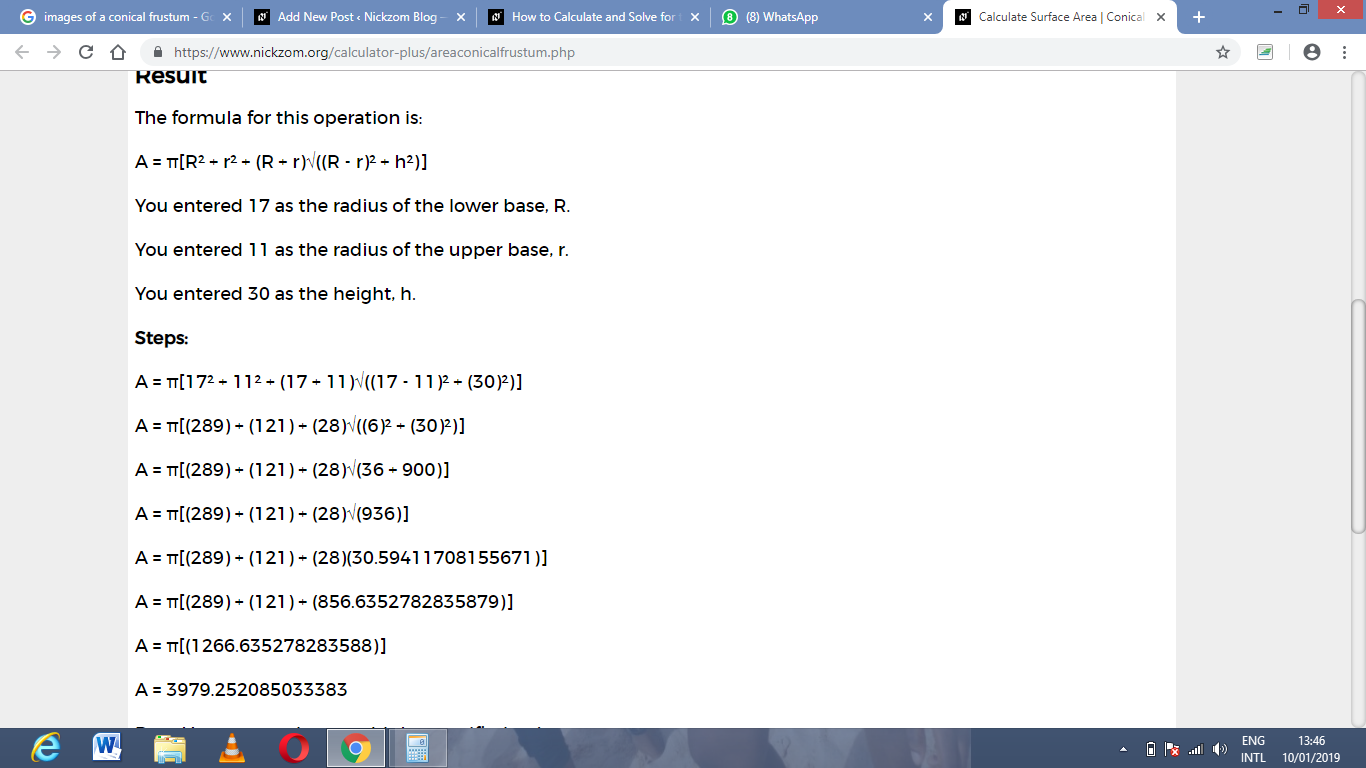As you can see from the screenshot above, Nickzom Calculator – The Calculator Encyclopedia solves for the Total Surface area of a conical frustum and presents the formula, workings and steps too.